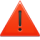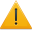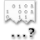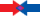Basic LuaThis page has multiple issues.See the Page Details section for information.

Variables

Variables provide a way to store and retrieve data for later use and are essential for any Lua script.

The Basics

This is the basic syntax for setting a variable:

<varname> = <value>

<varname> is the name of the variable you are using, or the identifier.
<value> is the data the variable will hold.

Here are some examples:

//creating global variables
IsFlying = false
VehicleName = "Jeep"
TotalFootsteps = 1337

//creating local variables
local IsNotFlying = true
local VehicleSpeed = "fast"
local TotalNonFootsteps = 7331

You can also assign the value of one variable to another variable like so:

Variable1 = "String!"
Variable2 = Variable1

Variable2 now holds the value "String!"

Some guidelines on variable naming:

• The name should be short, simple, and descriptive of what kind of data it holds.
• Be sure the name you use is unique or it may cause problems.
• Use locals whenever you can, using globals all the time can cause big problems. more info: Scope

Data Types
In Lua there are only a few types of data

The special type nil is only used if there is no data.

Checking Data Types
If you ever need to check what type of data a variable is holding you can use the type() function:

type( false ) //returns "boolean"
type( "Jeep" ) //returns "string"
type( 1337 ) //returns "number"
type( TheCake ) //returns "nil" because TheCake isn't set
TheCake = "is a lie."
type( TheCake ) //returns "string" because we just set 'TheCake' to the string "is a lie."
• The type() function will always return a string
• The string will be one of the seven types mentioned above

Please note that 1337 and 13.37 are both considered a number in Lua. Though when something says to use a float it means it is expecting a number with a decimal point.

Using Variables

Using a variable is very easy, since every variable has a name all you need to do to use one is say its name.

Here I'm going to use the Msg() and tostring() functions to print our variables to the console

• The Msg() function prints a string to the console.
• The tostring() function tries to turn any data it is given into a string.

IsFlying = false
VehicleName = "Jeep"
TotalFootsteps = 1337

Msg( tostring( IsFlying ) .. "\n" )
Msg( VehicleName .. "\n") // we already know this is a string, no need to use tostring() here
Msg( tostring( TotalFootsteps ) .. "\n" )

Notice how I used .. "\n", this is so that each new message prints on a seperate line.

This very simple script outputs:

false
jeep
1337

Here's one more example:

TotalShots = 2318
Misses = 981

Hits = TotalShots - Misses

• Hits is now equal to the number 1337 because 2318 - 981 = 1337

Knowing this you can now move on to making functions, which are another essential tool in creating Lua scripts.

Functions

Functions are sections of code that perform a certain task. The main advantage in using functions is that their code can be executed multiple times, where it would otherwise have to be replicated several times within a script. Parameters is a way to pass a variable or value to the function.

function <name>(<parameters>)
<instructions>
end

or

<name> = function(<parameters>)
<instructions>
end

The following code defines a function called PrintMessage, with one parameter, message. This function prints the contents of "message" to the console. Such a section of code is called a definition.

function PrintMessage( message )
Msg( message .. "\n" )
end

Calling a function results in the code inside the function definition being executed. In this case, the function call:

PrintMessage( "Hello world!" )

This will print "Hello world!" to the console.

Note: It is not necessary to make a function that simple. This was used as an example.

Functions can also return a value:

return a + b
end

// number should equal 7 after this
number = addAB( 5, 2 )

Scope

Now that you know what a variable and a function is, it is time to talk about scope. Scope is what can access a variable.

local myLocalVariable = "Hello"

function myFunction()
local myVariable = 5 // myVariable is a local variable and will be destroyed when we leave this scope (at the next "end")

mySecondVariable = 200 // mySecondVariable does not have the local prefix and is therefore a global variable, this will never be deleted unless set to "nil"
// ... instructions ...
end

print( myVariable ) // Outputs: "nil"
print( mySecondVariable ) // Outputs: "200"
print( myLocalVariable ) // Outputs: "Hello"

myVariable was declared inside myFunction() as a local variable. When myFunction is run, the variable is created. When myFunction ends, the variable is destroyed and no longer exists.

mySecondVariable however will persist even after the function has ended because it doesn't have the local prefix, and can therefore be used anywhere, even in other files! You should always use local variables whenever possible to avoid filling Lua with variables that will never be used again.

myLocalVariable was declared before the function, and is not inside any loops, functions or if-statements, and is therefore local to the file. This means that myLocalVariable can be accessed from anywhere in this file, but not from outside it.

Conditionals

Conditional statements, or conditionals, are a way of responding differently based on the result of a comparison, called a conditional expression. The comparison is usually between variables, though this need not always be the case.This is Garry's Mod Lua syntax, for more information see Lua Differences.

Comparisons are performed through the relational operators. These are:

Relational Operators

• Equal to (==)
• Not equal to (~= or !=)
• Greater than (>)
• Greater than or equal to (>=)
• Less than (<)
• Less than or equal to (<=)

Several expressions can be combined through the use of logical operators.

Logical Operators

• Not (not or !)
• And (and or &&)
• Or (or or ||)

Now you know what the relational and logical operators are, here is how you use them. Our first example uses an if statement:

if <expression> then
<code>
end

If the expression evaluates to being true, then the code is executed. If it is found to be false, then the code is skipped, unless an else statement is found, in which case, that code is executed.

if angle == angleB then
// Run these instructions if
// angle is equal to angleB
end

Any one of the relational expressions can go between our two variables. What about the logical operators?

if angle != angleB then
// Run these instructions if
// angle is NOT equal to angleB
end

if angle == angleB && score == 10 then
// Run these instructions if
// angle and angleB are equal,
// AND if score equals 10.
end

Note: 0 != false

Those with experience in other programming languages may expect 0 to evaluate as false. This is not so in Lua, where all numbers evaluate as true.

Loops

Loops are similar to an if condition, but the code will keep executing while the expression is true. There are two types of loops: for-loops, and while-loops.

At any time in any loop you can use break to kill it and prevent any further code in the loop from being executed.

For Loops

These types of loops can take on two basic structures. The Numeric-For and the Generic-For.

Numeric-For Loops

This is the basic structure for a numeric-for loop:

<code>
end

<var> is the name of a new variable to use for iterating and is first set to value <value>.
<condition> must be an integer or a statement equating to an integer.
<additive> is how much to increment the variable <var> the next time the loop is run.

• If <additive> is negative then <var> must be less-than or equal-to <condition> to stop the loop.
• If <additive> is positive then <var> must be greater-than or equal-to <condition> to stop the loop.
• If <additive> is 0 then <var> must be set to the value of <condition> manually to break the loop, otherwise it will continue forever.
• <additive> can be left out and Lua will use the integer 1 by default

For example:

for var=0, 5, 1 do
print( var .. "\n" )
end

for var=0, 5 do
print( var .. "\n" )
end

will both return:

0
1
2
3
4
5

While

for var=0, 5, 2 do
print( var .. "\n" )
end

will return:

0
2
4

Generic-For Loops

Next is the generic-for statement. The generic-for statement works over functions, called iterators. On each iteration, the iterator function is called to produce a new value, stopping when this new value is nil.

This is the basic structure for a generic-for:

for <var-list> in <exp-list> do
<code>
end

<var-list> is a list of one or more variable names separated by commas.
<exp-list> is a list of one or more expressions. However, usually the expression list only contains one element, a call to an iterator.

The most common use of generic-for loops is to iterate over a table:

To iterate over a table with only numerical keys use the ipairs iterator function.

mytable = {"one", "two", "three"}
for i, v in ipairs(mytable) do
print(i, v)
end

which outputs:

1	one
2	two
3	three

For a table with mixed key types use the pairs iterator function.

mytable = {key1 = "value1", key2 = "value2", key3 = "value3"}
for key, value in pairs(mytable) do
print(key, value)
end

which outputs:

key1	value1
key2	value2
key3	value3

This next example shows a mix of integer and non-integer keys

a = {}
a["first_key"] = "one"
a["second_key"] = 2
a["first"] = "one"
a["second"] = "two"
a["third"] = "three"
a = "three"
a["somekey"] = "something"
for i, v in pairs(a) do
print(i, v)
end

This example outputs:

3		three
first		one
first_key	one
second		two
second_key	2
somekey		something
third		three

Note that the keys aren't sorted alphabetically.

It is possible to create your own iterator functions, however, that is beyond the scope of this basic intro. For more information, read chapter 7 of the online "Programming in Lua" book found here: http://www.lua.org/pil/index.html#7

While Loops

A while loop is useful if you don't need an iterating value but still need to perform some code multiple times.

This is the basic structure for a while loop:

while <condition> do
<code>
end

<condition> should equate to either true or false.
A while loop will continue to execute until <condition> returns false

Some examples:

This loop will continue forever

while true do
print("Infinite loop!")
end

This loop will stop itself after 1.5 seconds

local starttime = os.clock()
print("I'm about to spam you horribly for 1.5 seconds")
while os.clock() - starttime < 1.5 do
print("SPAM!")
end
print("All done :P")

Repeat Loops

Repeat loops are very similar to while loops in that the condition must return true to end the statement. Think of it as the backwards cousin to the while loop.

This is the basic structure for a repeat loop:

repeat
<code>
until <condition>

<condition> should equate to either true or false.
A repeat loop will continue to execute until <condition> returns true

Here are the same examples from the while loop section but using a repeat loop instead.

This loop will continue forever

repeat
print("Infinite loop!")
until false

This loop will stop itself after 1.5 seconds

local starttime = os.clock()
print("I'm about to spam you horribly for 1.5 seconds")
repeat
print("SPAM!")
until os.clock() - starttime > 1.5
print("All done :P")

Tables

Tables are hashes that map keys to values. Keys and values can be any datatype, but it is important to remember that when using garbage collected keys, those keys are stored by reference; in short, using another table or, for example, a vector, as a key will not work like you expect it to. Tables can act as arrays by using numerical keys. You can access values in a table using the traditional [] syntax, or using the dot notation used in other languages to access fields of a structure. For example, table["string"] == table.string.

Syntax

Using a table constructor:

my_table = { first_key = 2, second_key = 5,  = 3 }

Creating an array, where numerical indices are automatically assigned (note that array indices start at 1):

my_table = { first, second, third }

When using the dot notation, first make sure that the table exists already! Otherwise, Lua thinks you are trying to index a nil value, since the table does not exist yet. Either using the table constructor, or bracket notation will suffice to tell Lua that it's a table. Also, note that numerical keys, or string keys beginning with a number will not work using dot notation. Using the dot notation:

my_table = {} // We must initialize the table before using dot notation!
my_table.first_key = 1
my_table.second_key = 2
/* note that when using dot syntax, you cannot use a number as a key,
or at the beginning of a string key!

ie:
my_table.3 = "string3" will NOT work
my_table.3string will NOT work

my_table.string3 WILL work
my_table["3string"] WILL work
*/

Using C style bracket [] syntax:

my_table = 1
my_table["two"] = 2  // note that strings and numbers
// can be used as keys in this syntax
my_table = "string3"

Two dimensional table:

my_table = {} // Initialize the first dimension of the table

// Now we can use
my_table = "hey"
my_table = 182

// But we CAN'T do this, because this index isn't assigned to a table,
// giving a nil error
my_table = 182

// So we need another table inside our table, so we do this
my_table = {} // This will make our third index a table, that means
// we can only use table functions on THIS index ONLY

// Now it will work fine!
my_table["hay"] = "sup"
my_table = 63

// Here's a summary on what it could look like:
my_table = {}

my_table = {}
my_table = "I'm a 2"
my_table = "dimensional"
my_table = "table!"

my_table = "not a 2D table"

// The above example can also we written as:
my_table= {{"I'm a 2", "dimensional", "table!"}, "not a 2D table :("}

What people do wrong:

// This is something many people do wrong
my_table = {{}} // This does NOT set up a 2D table on all indexes.
// So the code below would NOT work
my_table = "this"
my_table["thing"] = "code"
my_table["hai"] = "fails"

// The only this it does is making the first index a table!
// So that means it's the same as doing this:
my_table = {}
my_table = {} // Only the first index will be a 2D table

// So to declare the first 3 indexes, you need to do:
my_table = {{}, {}, {}}

// Which is the same as:
my_table = {}
my_table = {}
my_table = {}
my_table = {}

Example

Another good resource is the online "Programming in Lua" book: http://www.lua.org/pil/index.html

Page DetailsThis article is a stub.You can help the GMod Wiki by expanding it.This article has been tagged to be merged with Lua Tutorial Series. If you wish for the page to be handled differently, this can be disputed on the talk page. See more articles tagged to be merged here.Comments: None given.